# LightOJ1052 String Growth[矩阵快速幂]

## F - Problem F

LightOJ - 1052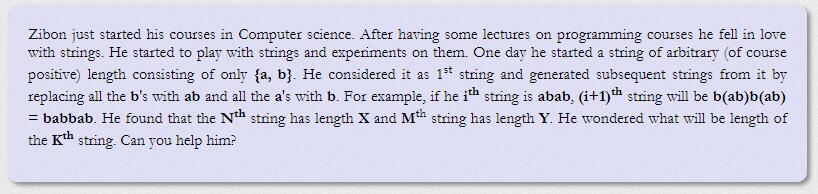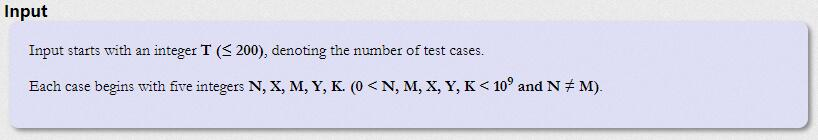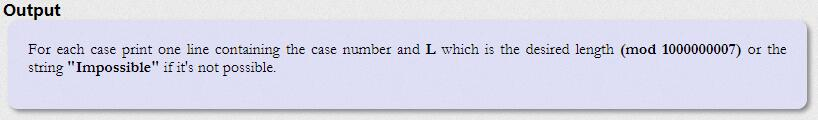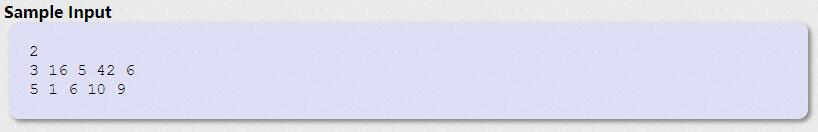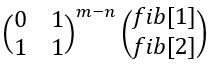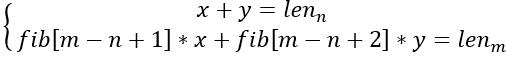之后我们就可以得到这两条式子。

① n>=45

② n==1

③ n>1 && n<45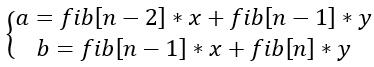不需要管 n 具体代指什么， 我们只是表达出他们的关系。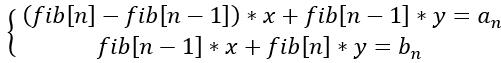之后会得到这样两条式子，继续解方程。(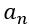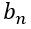分别代指第 n 个字符串的 a 的个数和 b 的个数，x，y 分别代指第 1 个字符串的 a 的个数和 b 的个数)

#pragma comment(linker, "/STACK:102400000,102400000")
#include<iostream>
#include<cstdio>
#include<cstring>
#include<cmath>
#include<string>
#include<algorithm>
#include<queue>
#include<stack>
#include<set>
#include<map>
#include<vector>
using namespace std;
typedef long long ll;
const int mod=1e9+7;
const int N=3;
ll aa[N][N],bb[N][N],ans[N][N],tmp[N][N];
void initial()
{
aa=0;
aa=aa=aa=1;
memset(ans,0,sizeof(ans));
for (int i=0 ; i<N ; ++i)
ans[i][i]=1;
}
void Pow(ll a[][N],ll b[][N],int n)
{
memset(tmp,0,sizeof(tmp));
for (int i=0 ; i<n ; ++i)
for (int j=0 ; j<n ; ++j)
for (int k=0 ; k<n ; ++k)
tmp[i][k]=(tmp[i][k]+a[i][j]*b[j][k]%mod)%mod;
for (int i=0 ; i<n ; ++i)
for (int j=0 ; j<n ; ++j)
a[i][j]=tmp[i][j];
}
void Pow(ll a[][N],ll m)
{
while (m)
{
if (m&1)
Pow(ans,a,2);
Pow(a,a,2);
m>>=1;
}
}
void getans(ll x,ll y,int p)
{
initial();
Pow(aa,p-1);
ll k1=(ans+ans)%mod;
ll k2=(ans+ans)%mod;
ll ans=(x*k1%mod+y*k2%mod)%mod;
printf("%lld\n",ans);
}
bool check(int x1,int y1,int n,int p)
{
if (n>=45)
return 0;
if (n==1)
{
if (x1+y1<=0)
return 0;
getans(x1,y1,p);
return 1;
}
initial();
Pow(aa,n-2);
ll k1=ans+ans;
ll k2=ans+ans;
ll x=(y1*k1-x1*k2)/(k1*k1-(k2*k2-k1*k2));
ll y=(y1-k1*x)/k2;
if (x<0 || y<0)
return 0;
getans(x,y,p);
return 1;
}
int main()
{
int T;
scanf("%d",&T);
for (int test=1 ; test<=T ; ++test)
{
int n,m,x,y,k;
scanf("%d%d%d%d%d",&n,&x,&m,&y,&k);
if (n>m)
{
swap(n,m);
swap(x,y);
}
printf("Case %d: ",test);
if (m-n<45)
{
ll a=1,b=1,c;
for (int i=0 ; i<m-n ; ++i)
{
c=a+b;
a=b;
b=c;
}
ll x1=b*x-y;
ll y1;
if (!(x1%(b-a)))
{
x1=x1/(b-a);
y1=x-x1;
if (x1<0 || y1<0 || !check(x1,y1,n,k))
printf("Impossible\n");
}
else
printf("Impossible\n");
}
else
printf("Impossible\n");
}
return 0;
}